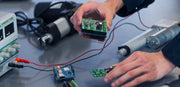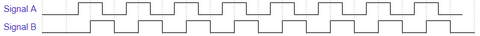# How to read the signal from a hall effect sensor using an Arduino?PA Engineer

Hall effect sensors are one of the feedback options we offer in our linear actuators. Our PA-04-HS and PA-100 have Hall effect sensors on the stock units. While several of our other actuators can be custom ordered with Hall effect sensors.

## What is a Hall effect sensor?

A Hall effect sensor is an electronics component that generates a voltage based on the strength of the magnetic field flowing through it. When this sensor is paired with voltage threshold detecting circuitry a signal with two states can be produced. In our actuators, the hall effect sensor is designed to produce two signals in either of two binary states; on, or off. These two signals will rise and fall as the electric motor rotates with a 90° phase difference between them, as shown below.## How do you read these signals?

When reading a digital signal on a microcontroller there are two main methods; polling, and interrupts. Polling is a programmed method in which a microcontroller periodically checks the state of an input to see if there has been a change. While interrupts are a hardware mechanism that immediately shifts the focus of the microcontroller’s program when the state changes on an input. Each of these methods has its pros and cons, and each has applications that they are better suited for. For our case, we will want to know the exact moment when a signal changes state, so we will be using interrupts. On an Arduino microcontroller, an interrupt is used by creating an ISR or Interrupt Servicing Routine, an example of this is shown in the code below.

 // global volatile variables are needed to pass data between the // main program and the ISR's volatile byte signalA; volatile byte signalB; // the pins that can be used with interrupts depend on the board you // are using const byte inputA = 2; const byte inputB = 3; void setup() {   // enable internal resistors on the input pins   pinMode(inputA, INPUT_PULLUP);   pinMode(inputB, INPUT_PULLUP);   // read the initial state of the inputs   signalA = digitalRead(inputA);   signalB = digitalRead(inputB);   // will detect a rising or a falling edge   attachInterrupt(digitalPinToInterrupt(inputA),signalA_ISR,CHANGE);   attachInterrupt(digitalPinToInterrupt(inputB),signalB_ISR,CHANGE); } void loop() {   // This is where the signal information can be used in a program } void signalA_ISR() {   // when a change is detected it will always be   // to the opposite of the current state   signalA = !signalA; } void signalB_ISR() {   signalB = !signalB; }

## What needs to be considered in the application?

Since the signals we are reading are going to be high frequency there are a few considerations that need to be made. Firstly, how long will the program take to execute the ISR? How many separate signals need to have ISR’s? How fast is the microcontroller’s clock speed?

A problem that can be encountered in a program with a lengthy ISR is that the ISR will be triggered again before it has completed the code it contains from the previous time it was triggered. It is recommended to keep the minimum amount of code in an ISR to help avoid this problem.

In the example code above, two signals are set up with interrupts. Both signals are needed to be able to detect the direction of the movement of the linear actuator. The downside to enabling interrupts on both signals is that an ISR is triggered twice as often. In applications where the direction of the movement of the actuator is not needed to be known or is already apparent from the program being run, only one signal would need an interrupt.

Some microcontrollers have the ability to use faster or slower clock speeds. The clock speed changes how fast the microcontroller can run the program. If the frequency of the signals being read is high, then the clock speed may have to be increased. However, it is the most power-efficient to use as slow of clock speed as the application allows.

## What if the microcontroller is not fast enough?

After the above considerations have been made, sometimes the microcontroller is just not fast enough to get through the main code and keep up with the ISR’s. In these cases, you may want to make use of an additional microcontroller. One microcontroller can be used to run the ISR’s, read the data, then transmit the data needed to another microcontroller where the main code can be executed without being interrupted.

## What information can the signals provide?

While the Hall effect sensors in our actuators only provide two binary signals, the signals themselves can be used in a program to calculate several different useful parameters.

• Direction of motion.
• Position of the actuator rod.
• The velocity of the actuator rod.

### Direction

The direction of the movement of an actuator can be determined by looking at the current state of the two signals and comparing it to the last state of the two signals. This is because the two signals will change which is leading and which is lagging depending on the direction of the movement of the actuator.

 Current edge detected Last edge detected Direction Signal A rising Signal B falling Retracting Signal A falling Signal B rising Retracting Signal A rising Signal B rising Extending Signal A falling Signal B falling Extending

### Position

The position of the actuator requires some calculations using the hall effect signals and some measurements of the actuator itself. The equation for the position of the actuator rod requires the stroke length of the actuator, and the total number of edges detected from fully retracted to fully extended. Using these measurements the following equation can be used:The edges detected since fully retracted in the above equation will start at zero and increase by one when an edge is detected in the forward direction and decrease by one when an edge is detected in the backward direction.

### Velocity

The velocity of an actuator can be measured using the Hall effect signals by implementing a timer in the program. This timer will be used to measure the time between edges detected. Additionally, the calculated value of the change in stroke per edge detected is required. Using these values the following equation can be used: